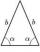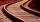# Angle + real numbers - math problems

#### Number of problems found: 13

• CotangentIf the angle α is acute, and cotan α = 1/3. Determine the value of sin α, cos α, tg α.
• CuboidDetermine the dimensions of cuboid a, b, c; if diagonal d=9 dm has angle with edge a α=55° and has angle with edge b β=58°
• BuildingHow high is the building that throws horizontal shadow 95.4 m long at angle 50°?
• ObserverThe observer sees a straight fence 100 m long in 30° view angle. From one end of the fence is 102 m. How far is it from another end of the fence?
• Triangle TBCTBC is isosceles triangle with base TB with base angle 63° and legs length |TC| = |BC| = 25. How long is the base TB?
• Trigonometric functionsIn the right triangle is: ? Find the value of s and c: ? ?
• Rail turnThe curve radius on the railway line is 532 m . Length of rail track on it is 818 m. How many degrees is the angle ASB, if A and B are boundary points and S is center of arc curve.
• Cone A2VThe surface of the cone in the plane is a circular arc with central angle of 126° and area 415 cm2. Calculate the volume of a cone.
• SlopeWhat is the slope of a line with an inclination 6.06 rad?
• Regular 5-gonCalculate the area of the regular pentagon with side 7 cm.
• IS triangleCalculate interior angles of the isosceles triangle with base 40 cm and legs 22 cm long.
• Quarter circularThe wire that is hooked around the perimeter of quarter-circular arc has length 3π+12. Determine the radius of circle arc.
• AnglesDetermine the interior angles of a rhombus with area 319.1 cm2 and perimeter 72 cm.

We apologize, but in this category are not a lot of examples.
Do you have an interesting mathematical word problem that you can't solve it? Submit a math problem, and we can try to solve it.

We will send a solution to your e-mail address. Solved examples are also published here. Please enter the e-mail correctly and check whether you don't have a full mailbox.

Please do not submit problems from current active competitions such as Mathematical Olympiad, correspondence seminars etc...

Angle Problems. Real numbers - math problems.